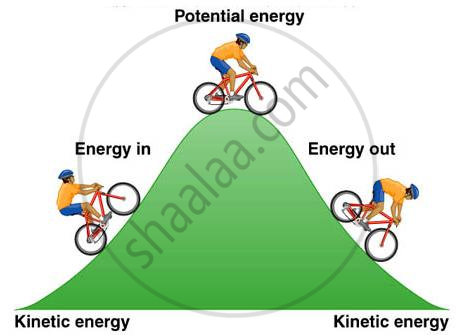Share

# Work and Energy - Potential Energy

Course

#### description

• Expression for potential energy

#### notes

Potential Energy:

Every object possesses some energy called Potential Energy. An object when gains energy may store it in itself as potential energy.We know that when an object rises above the ground some work is done against gravity. Since work is done on the object, the object would gain some energy. The energy that the object gains at a height is called Gravitational Potential Energy. It is defined as the amount of work done required in raising an object above the ground up to a certain point against the gravity.

W = F x d = F x h (height)

And F = m x g (because the force is applied against gravity)

So, W = m x g x h

Hence potential energy of object A, Ep = m x g x h

Elastic Potential energy: Same work has to be done to change the shape of a body. This work gets stored in the deformed body in the form of elastic potential energy. Elastic potential energy is never negative whether due to extension or to compression.

### Shaalaa.com

Potential Energy [00:07:03]
S
series 1
0%

S